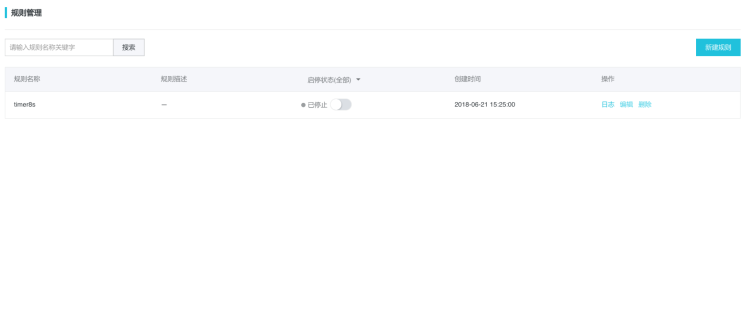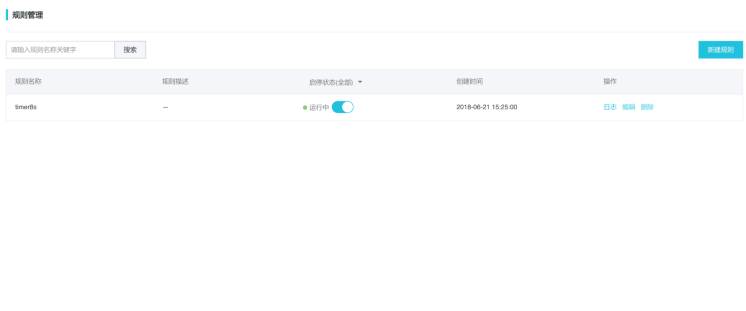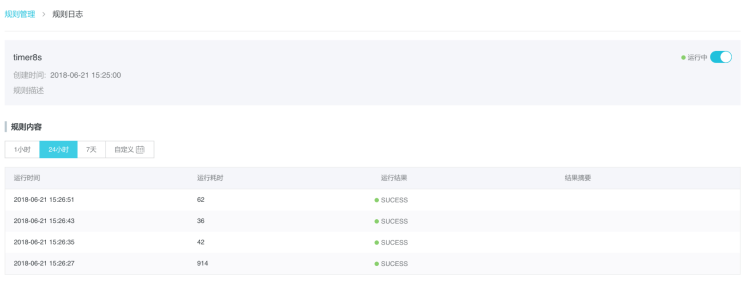新建规则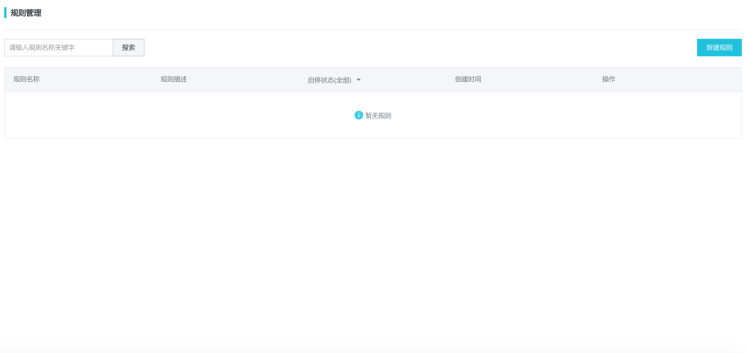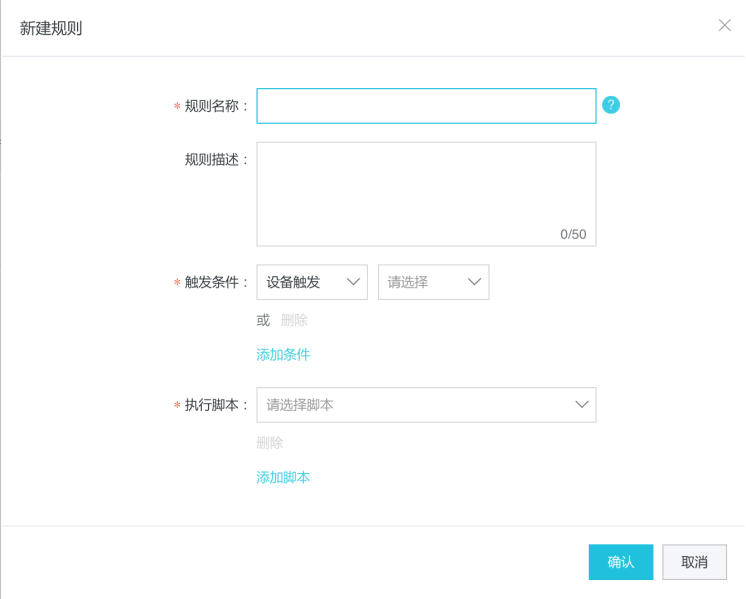设备触发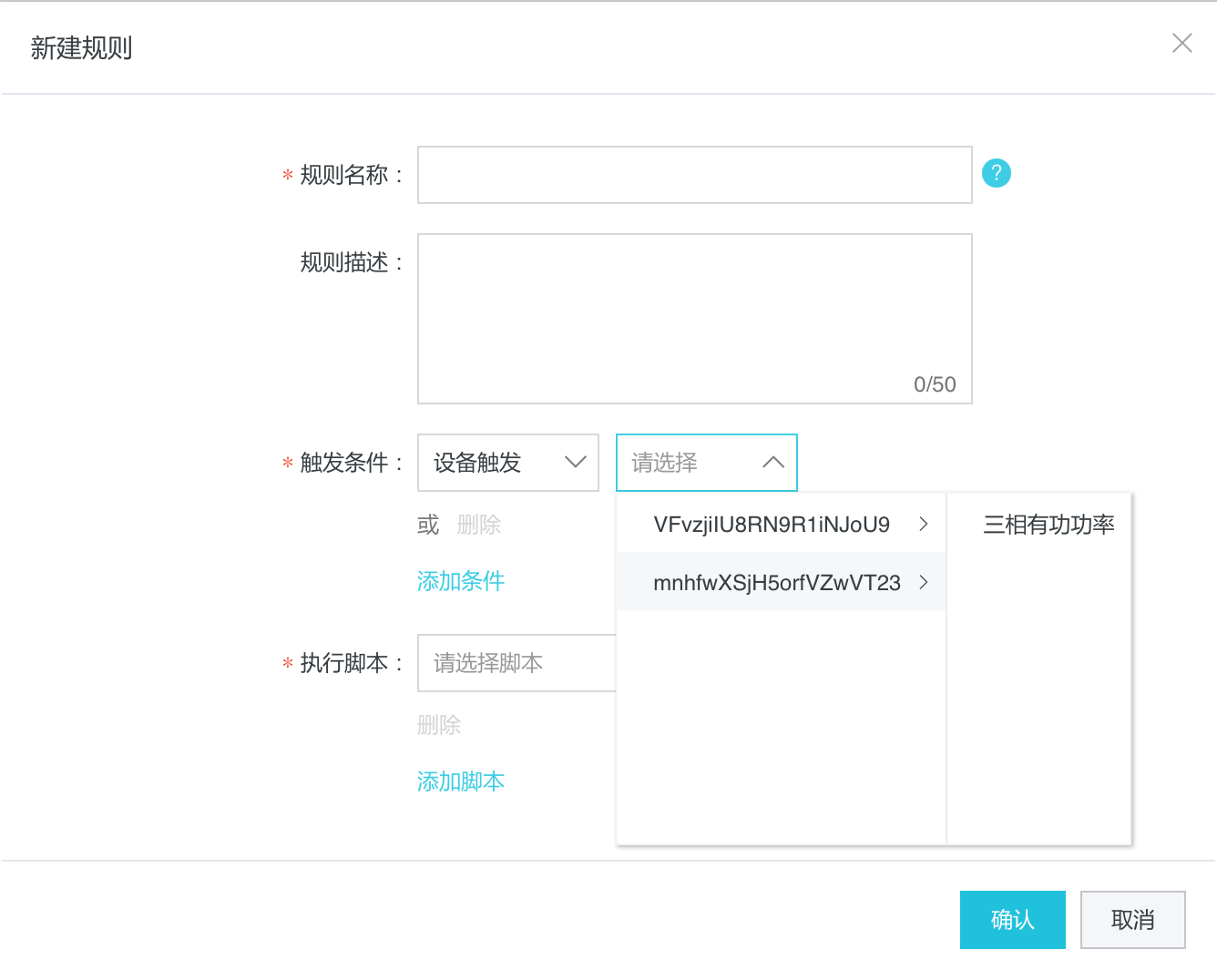属性类型 条件 值 字符串 =,!=,like,in,not like 字符串 整型 =,!=,>,<,>=,<=,in,between 整型 浮点型 =,!=,>,<,>=,<=,in,between 浮点型 枚举型 =,!=,>,<,>=,<=,in,between 整型 布尔型 =,!= true或false 时间型 >,>=,<=,==,!= 日期

定时触发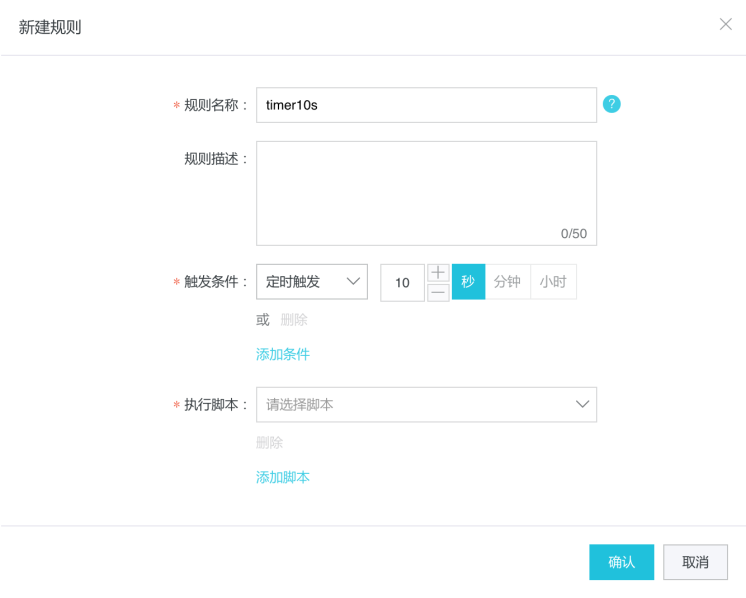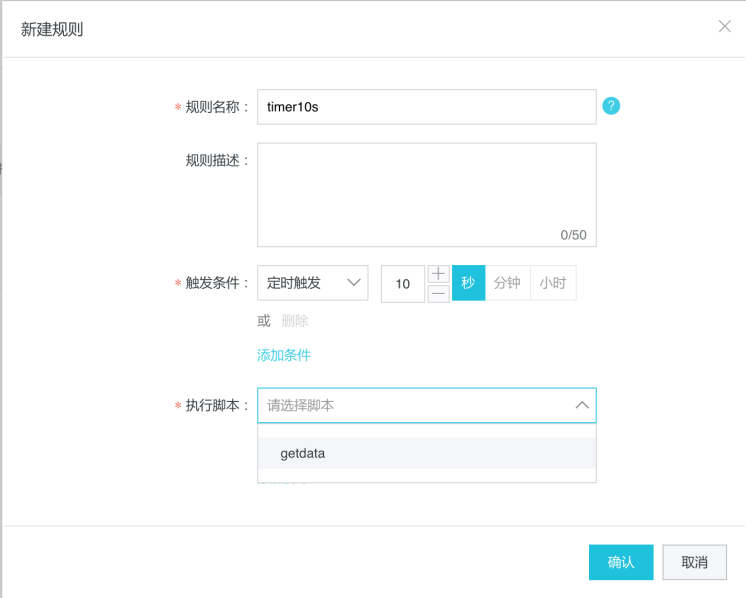规则启停